## 11.5.3 Examples with Nature Sensing Actions

This section illustrates the effect of nature sensing actions, but only for the nondeterministic case. General methods for computing probabilistic I-states are covered in Section 11.6.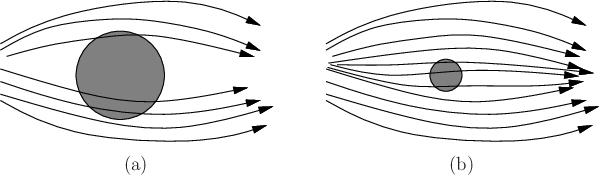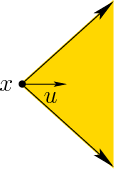Example 11..22 (Above or Below Disc?)   This example involves continuous time. Suppose that the task is to gather information and determine whether the state trajectory travels above or below some designated region of the state space, as shown in Figure 11.23.

Let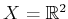. Motions are generated by integrating the velocity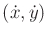, which is expressed as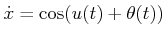and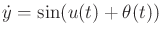. For simplicity, assume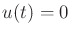is applied for all time, which is a command to move right. The nature action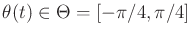interferes with the outcome. The robot tries to make progress by moving in the positive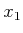direction; however, the interference of nature makes it difficult to predict the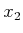direction. Without nature, there should be no change in thecoordinate; however, with nature, the error in thedirection could be as much as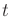, afterseconds have passed. Figure 11.24 illustrates the possible resulting motions.

Sensor observations will be made that alleviate the growing cone of uncertainty; use the sensing model from Figure 11.11, and suppose that the measurement error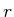is. Suppose there is a disc in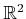of radius larger than, as shown in Figure 11.23a. Since the true state is never further thanfrom the measured state, it is always possible to determine whether the state passed above or below the disc. Multiple possible observation histories are shown in Figure 11.23a. The observation history need not even be continuous, but it is drawn that way for convenience. For a disc with radius less than, there may exist some observation histories for which it is impossible to determine whether the true state traveled above or below the disc; see Figure 11.23b. For other observation histories, it may still be possible to make the determination; for example, from the uppermost trajectory shown in Figure 11.23b it is known for certain that the true state traveled above the disc.Example 11..23 (A Simple Mobile Robot Model)   In this example, suppose that a robot is modeled as a point that moves in. The sensing model is the same as in Example 11.22, except that discrete stages are used instead of continuous time. It can be imagined that each stage represents a constant interval of time (e.g.,second).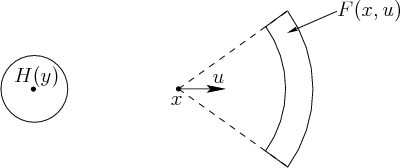To control the robot, a motion command is given in the form of an action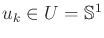. Nature interferes with the motions in two ways: 1) The robot tries to travel some distance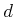, but there is some error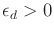, for which the true distance traveled,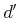, is known satisfy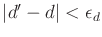; and 2) the robot tries to move in a direction, but there is some error,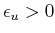, for which the true direction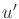is known to satisfy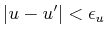. These two independent errors can be modeled by defining a 2D nature action set,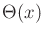. The transition equation is then defined so that the forward projection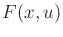is as shown in Figure 11.25.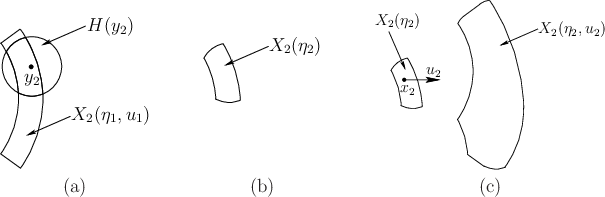Some nondeterministic I-states will now be constructed. Suppose that the initial stateis known, and history I-states take the form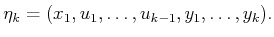(11.73)

The first sensor observation,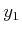, is useless because the initial state is known. Equation (11.29) is applied to yield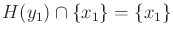. Suppose that the action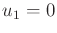is applied, indicating that the robot should move horizontally to the right. Equation (11.30) is applied to yield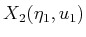, which looks identical to theshown in Figure 11.25. Suppose that an observation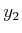is received as shown in Figure 11.26a. Using this,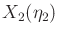is computed by taking the intersection of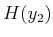and, as shown in Figure 11.26b.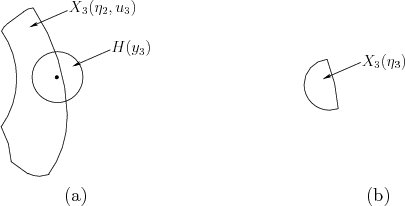The next step is considerably more complicated. Suppose that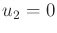and that (11.30) is applied to compute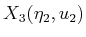from. The shape shown in Figure 11.26c is obtained by taking the union of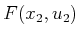for all possible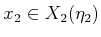. The resulting shape is composed of circular arcs and straight line segments (see Exercise 13). Once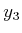is obtained, an intersection is taken once again to yield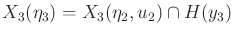, as shown in Figure 11.27. The process repeats in the same way for the desired number of stages. The complexity of the region in Figure 11.26c provides motivation for the approximation methods of Section 11.4.3. For example, the nondeterministic I-states could be nicely approximated by ellipsoidal regions.Steven M LaValle 2020-08-14